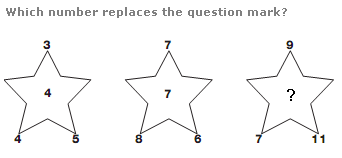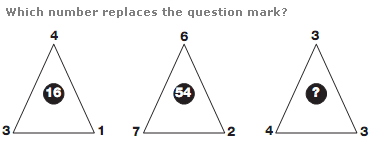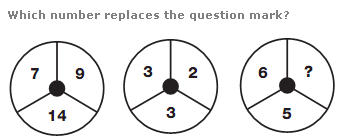# Puzzles - Number puzzles

### Exercise :: Number puzzlesAnswer : 9 Explanation : In each star, the average of the outer 3 numbers is written in the centre of the star.Answer : 21 Explanation : In each triangle, add the lower 2 digits together and multiply by the upper digit to give the result in the centre.Answer : 1 Explanation : In each circle, add together the numbers in the left and right hand segments, and subtract 2 to give the result in the lower segment.